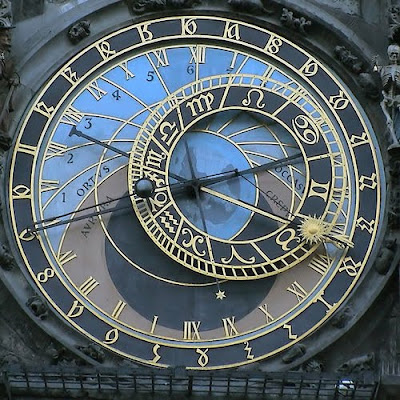## Friday, June 11, 2010

Each pixel is blended with fog color. The bigger pixel distance from observer location - the more pixel color approaches fog color. GLSL fragment code:

`uniform sampler2D tex;void main(){ float FogDensity = 10.; vec4 FogColor = vec4(0.4,0.2,0.2,1.0); vec4 CurrentColor = texture2D(tex, gl_TexCoord.xy);  // distance to target float FogDistance = distance(vec2(0.49,0.46),gl_TexCoord.xy);  // fog factor float FogFactor = exp(-abs(FogDistance * FogDensity)); // linear blend between fog color and pixel color gl_FragColor = mix(FogColor,CurrentColor,FogFactor);}`

Image